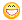About Store Forum Documentation ContactGame::Item pos/matrix functions Author Message
krokodilcapaMember

 Post: #1 Game::Item pos/matrix functions Hi! Somebody could give me the Game::Item class's pos,matrix and matrixScaled function's codes (get and set too)? Of course if its not problem, because I'm not purchased the Game objects sources.
03-01-2013 05:11 PM
RubeusMemberPost: #2 RE: Game::Item pos/matrix functions It's in the header: // get / set virtual Vec pos ( ); // get position virtual void pos (C Vec &pos ); // set position virtual Matrix matrix ( ); // get matrix , returned matrix is normalized virtual Matrix matrixScaled( ); // get matrix , returned matrix is scaled by 'scale' virtual void matrix (C Matrix &matrix); // set matrix , 'matrix' must be normalized Keep in mind that Game::Item is meant to be inherited.
03-01-2013 05:30 PM
krokodilcapaMember

 Post: #3 RE: Game::Item pos/matrix functions Okay, then I write my problem: I've created my own inherited class based on Game::Obj. So I have to set all of these get/set parameters for matrix and position (because in base Game::Obj its all NULL). The position get/set is works great, I used my object's actor's position. But the matrix have one issue: if I load a world I created in the editor (I placed my object somewhere, not center), then the object is on the center of the world, same when I create it by code : Code: `Game::World.objCreate(*Game::Objs("myObj.obj"), Matrix(1, Vec(15,0,5));` my obj matrix set is also based on actor's matrix: Code: ```void MyClass::matrix(C Matrix &matrix) {     actor.matrix(matrix); }``` The most confusing thing is if I directly set the actor's matrix from code myobjs.actor.matrix(Matrix(1,Vec(5,0,5))); its works okay. Holy f*ck, problem solved. I was super careless, I forgot to set the matrix from the ObjParams at the create.But I still have the question, is it right if I use object matrix get/set simple from/to the object's actor matrix? (This post was last modified: 03-01-2013 07:52 PM by krokodilcapa.)
03-01-2013 07:36 PM
 « Next Oldest | Next Newest »